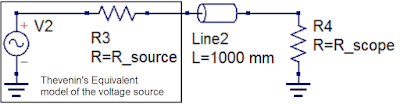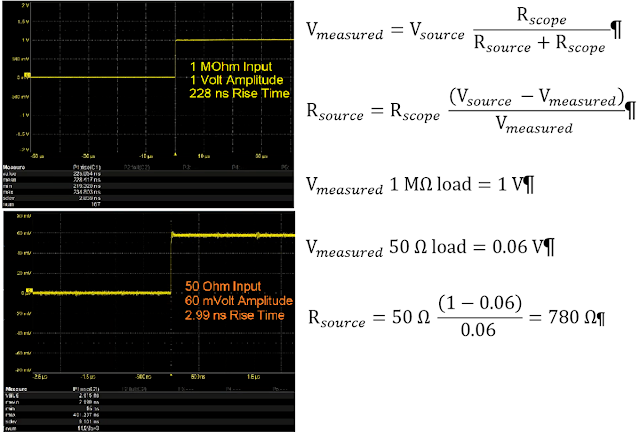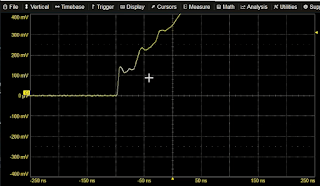## You need to test, we're here to help.

You need to test, we're here to help.

## 07 February 2022

### Transmission Lines for Oscilloscope Users, Part 3Figure 1: The Thevenin equivalent circuit model can be usedto characterize a voltage source with respect to the interconnect cable and oscilloscope input termination.
In Part 2, we demonstrated how to calculate the instantaneous impedance of a transmission line. However, any measurement made using an oscilloscope should consider not only the transmission line, but the source, the transmission line and the oscilloscope as a system. Therefore, characterizing your source, as well as knowing the effects of your oscilloscope input impedance, is important to developing the “situational awareness” needed to interpret measurements properly.

Two terms needed for us to characterize the source are the Thevenin source voltage and the Thevenin source resistance. Once we know these, we have all the pieces we need to fully understand what is happening with our measurements. This is true whether the signal source is a Cal terminal or a device-under-test.

Every voltage source to first order can be described as a simple Thevenin model (Figure 1). The Thevenin equivalent circuit model for a voltage source is an ideal voltage source plus a series Thevenin or output resistance. The value of the output resistance can be determined from two simple oscilloscope measurements: the voltage amplitude with a high impedance load and the voltage amplitude with a 50 Ω load.

When we attach our 50 Ω oscilloscope input impedance to the Thevenin model source, we have built a voltage divider: the output impedance of the voltage source plus the input impedance of the oscilloscope. This reduces the ideal voltage source amplitude at the input of the oscilloscope. Simply connecting the transmission line to the oscilloscope applies a 50 Ω load.

The input impedance of our coaxial cable transmission line is 50 Ω. If we have a 3 ft cable, the time delay is 5 ns down, 5 ns back. So, for at least the first 10 ns, the incident signal is going to see 50 Ω instantaneous impedance at the input to the transmission line. However, because of the voltage divider, we don't see the full output voltage of the source launched into the transmission line.

When the oscilloscope input termination is set to 1 MΩ, regardless of what is the output resistance of the source, as long as it's really small compared to 1 MΩ, the output is effectively “open.”

There is a simple, two-step procedure for calculating the Thevenin source resistance.

Step 1 is to measure the source voltage with the oscilloscope set to 1 MΩ termination. If we wait long enough for reflections to die out, then the voltage measured with 1 MΩ oscilloscope input impedance should be equivalent to the Thevenin output voltage. The measured voltage (Vmeasured) of our Cal signal at 1 MΩ is 1 V, the open circuit voltage of the Thevenin source (Vsource.). Note the 228 ns rise time, same as we measured using the 3 ft cable in Part 1.

Step 2 is to change the oscilloscope input impedance to 50 Ω and repeat the voltage measurement. Note that the input voltage must be under 5 Vrms to do this, because the maximum power that can be dissipated by that 50 Ω termination resistor in the oscilloscope is about a half a watt. When the input termination is set to 50 Ω, the measured voltage (Vmeasured) is 60 mV, due to the voltage divider. With a little arithmetic, we can calculate the output impedance of the Cal signal source as 780 Ω. Note the measured rise time is now 2.99 ns, a far more reasonable number than the 228 ns originally measured.Figure 2: Calculating the Thevenin voltage and resistance of the Cal signal source.

We can then use the component values of the Thevenin source we just calculated to look in detail at why the signal rise time is so high when measured using a 1 MΩ terminated oscilloscope input. Given what we now know about how changes in impedance will cause reflections, we can surmise what happens when we measure the rise time with a 1 MΩ termination.

As the reduced amplitude signal propagates down the cable, when it hits the 1 MΩ impedance of the oscilloscope input, the 1 MΩ oscilloscope input reflects all the signal back toward the source. The reflection has an amplitude of 60 mV, which added to the incident 60 mV produces a 120 mV signal at the oscilloscope input. The 60 mV reflection returns to the cable input after 10 ns, where it is again reflected off the 780 Ω output impedance of the Cal source. This time the reflection amplitude is about 90% of the 60 mV signal as it returns to the oscilloscope input. Reflections continue back and forth until the reflection amplitude is reduced to zero.Figure 3: The oscilloscope initially sees the sum of the 60 mV incident signal andthe 60 mV reflection. Additional reflections slowly increase the signal amplitude at the oscilloscope input.
Figure 3 Shows the Cal signal measured over a 9 ft cable with the oscilloscope input termination set to 1 MΩ.

The delay for the 9 ft cable is 15 ns each way for a round-trip propagation delay of 30 ns. The first step shown in the trace on Figure 3 is the sum of the 60 mV incident signal and the 60 mV reflection. 30 ns later, a step of about 108 mV bumps the voltage higher. Every 30 ns thereafter, the voltage steps up in a slowly decreasing amplitude until the reflections die out.

The longer rise times we saw with increased cable lengths are due to these multiple reflections. Changing the cable length increases the time between reflections and makes the measured rise time a function of cable length. If we terminate the cable by selecting a matching oscilloscope input termination of 50 Ω, the reflections are eliminated and the rise time is correctly measured as 2.99 ns, as shown in Figure 2. This is the rise time of the Thevenin source.

In our next post, we'll look at a waveform that is another "fingerprint" of reflections due to impedance mismatches.

Watch Dr. Eric Bogatin explain these basic principles of signal integrity in our on-demand webinar, What Every Oscilloscope User Needs To know About Transmission Lines.

Ready to go beyond “Transmission Lines 101”? You’ll find “Transmission Lines 201” in our earlier series:

Transmission Lines, Part I

Transmission Lines, Part II